Related Articles
Image Processing without OpenCV | Python
• Difficulty Level : Expert
• Last Updated : 03 Apr, 2019

We know OpenCV is widely used to operate on images and has a wide spectrum of functions to do so. But what if we want to process the image files without using any external library like OpenCV. Let’s see how can we do this.

### Image Scaling (Using Nearest Neighbour Interpolation):

Nearest Neighbour interpolation is the simplest way of interpolation. This method simply determines the “nearest” neighboring pixel and assumes the intensity value of it.

Consider a small image which is ‘w’ pixels wide by ‘h’ pixels high, which we want to re-size to ‘p’ pixels wide by ‘q’ pixels high, assuming that p>m and q>n. Now, we need two scaling constants:

```scale_x = p/w
scale_y = q/h```

Now, we simply loop through all the pixels in the output image, addressing the source pixels to copy from by scaling our control variables by scale_x and scale_y, and rounding the resulting scaled index values.

Pictorial representation :
Image of 3X3 pixel (total 9 pixels), now if we want to increase the size of image up to 6X6 then, according to nearest neighboring algorithm 6/3 (i.e 2) pixels should have the same RGB value as that of the pixel in original Image.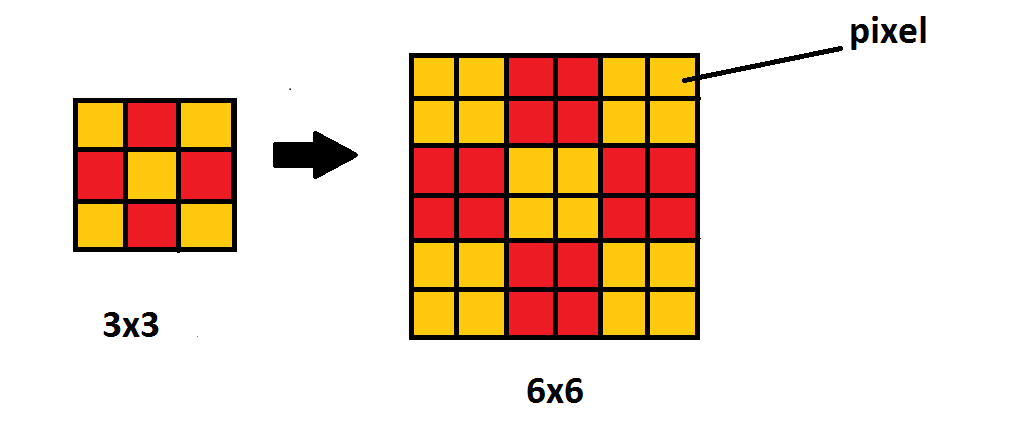Program for scaling an Image:

 `# using matplotlib and numpy`` ` `import` `matplotlib.image as img``import` `numpy as npy`` ` `# provide the location of image for reading ``m ``=` `img.imread(``"taj.png"``);`` ` `# determining the length of original image``w, h ``=` `m.shape[:``2``];`` ` `# xNew and yNew are new width and``# height of image required ``after scaling``xNew ``=` `int``(w ``*` `1` `/` `2``);``yNew ``=` `int``(h ``*` `1` `/` `2``);`` ` `# calculating the scaling factor ``# work for more than 2 pixel ``xScale ``=` `xNew``/``(w``-``1``);``yScale ``=` `yNew``/``(h``-``1``);`` ` `# using numpy taking a matrix of xNew``# width and yNew height with ``# 4 attribute [alpha, B, G, B] values``newImage ``=` `npy.zeros([xNew, yNew, ``4``]);`` ` `for` `i ``in` `range``(xNew``-``1``):``   ``for` `j ``in` `range``(yNew``-``1``):``       ``newImage[i ``+` `1``, j ``+` `1``]``=` `m[``1` `+` `int``(i ``/` `xScale),``                                 ``1` `+` `int``(j ``/` `yScale)]`` ` `# Save the image after scaling ``img.imsave(``'scaled.png'``, newImage);`

Output: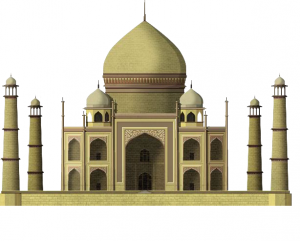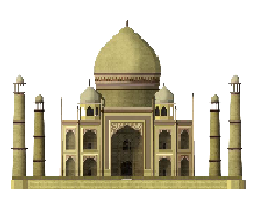### Gray Scaling of an Image:

Using the average value method, this method highlights the intensity of the pixel rather than showing what RGB values it consists. When we calculate the average value of RGB and assign It to RGB value of pixel, Since the RGB value of the pixel is same, it will not be able to create any color since all the colors are formed due different ratio of RGB value since in this case ratio will be 1:1:1. Hence Image then formed will look as gray Image.

Pictorial representation :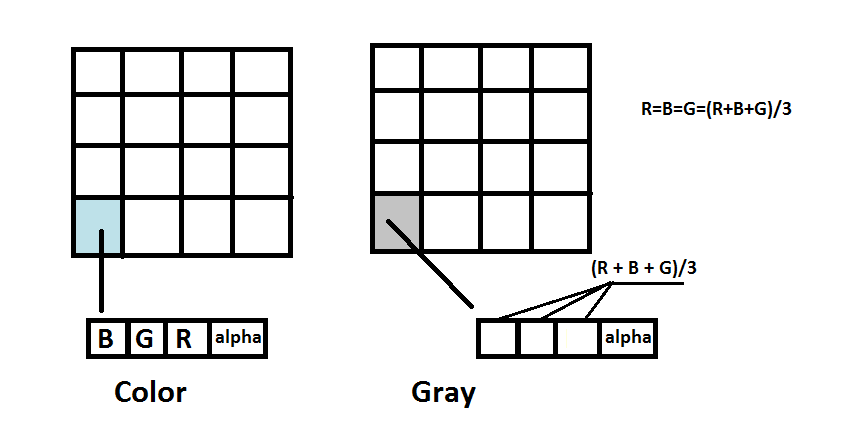Program for Gray scaling an Image :

 `# using numpy ``import` `numpy as npy`` ` `# using matplotlib``import` `matplotlib.image as img`` ` `# using statistics to import mean``# for mean calculation ``from` `statistics ``import` `mean `` ` `m ``=` `img.imread(``"taj.png"``)`` ` `# determining width and height of original image``w, h ``=` `m.shape[:``2``]`` ` `# new Image dimension with 4 attribute in each pixel ``newImage ``=` `npy.zeros([w, h, ``4``])``print``( w )``print``( h )`` ` `for` `i ``in` `range``(w):``   ``for` `j ``in` `range``(h):``      ``# ratio of RGB will be between 0 and 1``      ``lst ``=` `[``float``(m[i][j][``0``]), ``float``(m[i][j][``1``]), ``float``(m[i][j][``2``])]``      ``avg ``=` `float``(mean(lst))``      ``newImage[i][j][``0``] ``=` `avg``      ``newImage[i][j][``1``] ``=` `avg``      ``newImage[i][j][``2``] ``=` `avg``      ``newImage[i][j][``3``] ``=` `1` `# alpha value to be 1`` ` `# Save image using imsave``img.imsave(``'grayedImage.png'``, newImage)`

Output: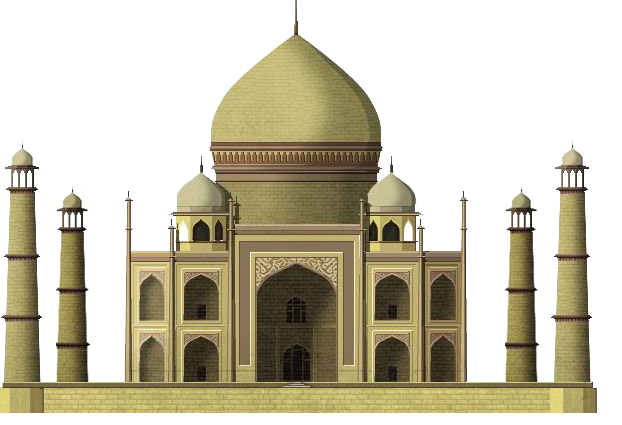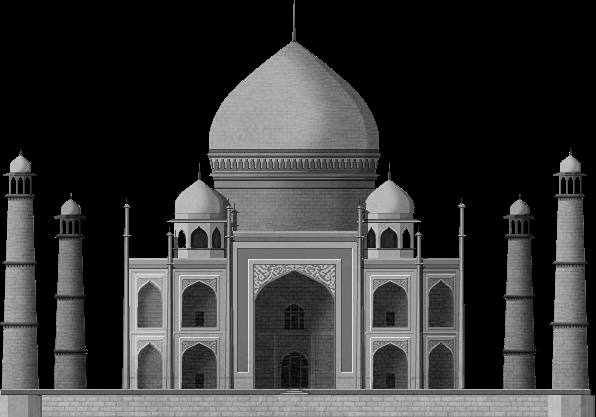### Cropping of an Image:

Cropping is basically removing the unwanted pixel. This can be done by taking required pixel in a different Image grid whose size is as required after cropping.

Consider an Image of size 10 X 10 pixel and if we require to crop only the center of image with 4 X 4 pixel then we need to collect the pixel values from (10-4)/2 starting from (3, 3) up to 4 pixel in x direction and 4 pixel in y direction.

Pictorial representation :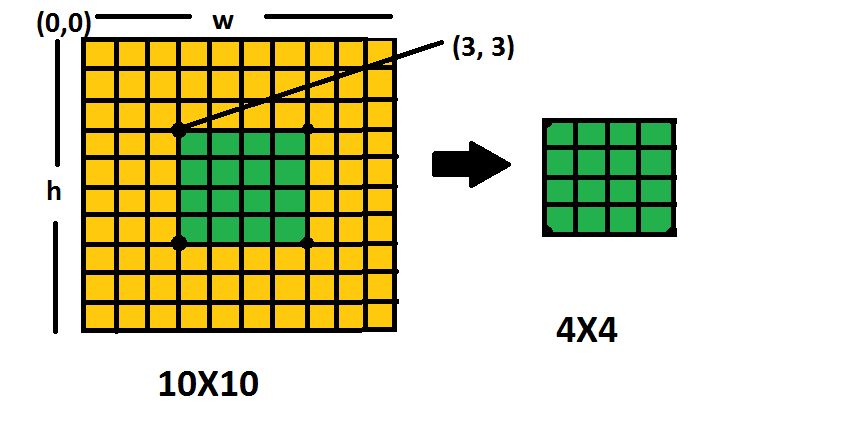Program for cropping an Image :
 `# using matplotlib and numpy``import` `matplotlib.image as img``import` `numpy as npy`` ` `# reading image in variable m``m ``=` `img.imread(``"taj.png"``)`` ` `# determining dimesion of image width(w) height(h)``w, h ``=` `m.shape[:``2``]`` ` `# required image size after cropping``xNew ``=` `int``(w ``*` `1` `/` `4``)``yNew ``=` `int``(h ``*` `1` `/` `4``)``newImage ``=` `npy.zeros([xNew, yNew, ``4``])`` ` `# print width height of original image``print``(w)``print``(h)`` ` `for` `i ``in` `range``(``1``, xNew):``    ``for` `j ``in` `range``(``1``, yNew):``       ``# cropping start from 100, 100 pixel of original image``        ``newImage[i, j]``=` `m[``100` `+` `i, ``100` `+` `j]`` ` `# save image ``img.imsave(``'cropped.png'``, newImage)`

Output: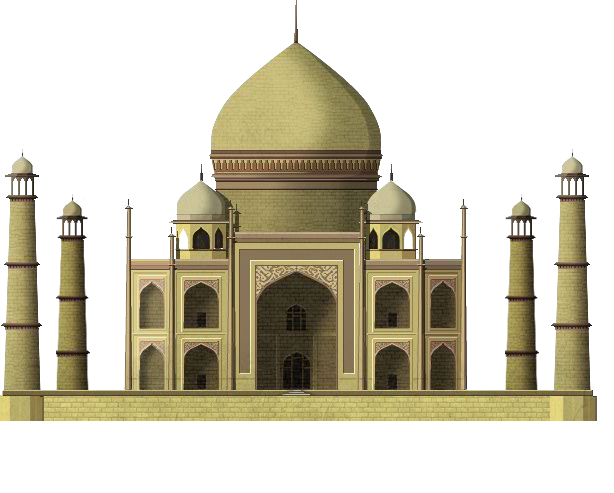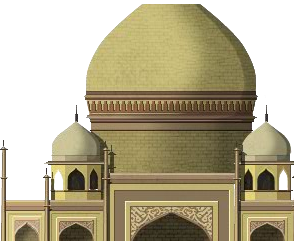Attention geek! Strengthen your foundations with the Python Programming Foundation Course and learn the basics.

To begin with, your interview preparations Enhance your Data Structures concepts with the Python DS Course.

My Personal Notes arrow_drop_up